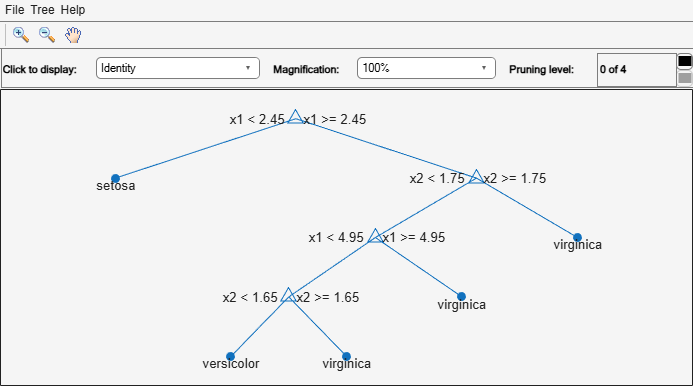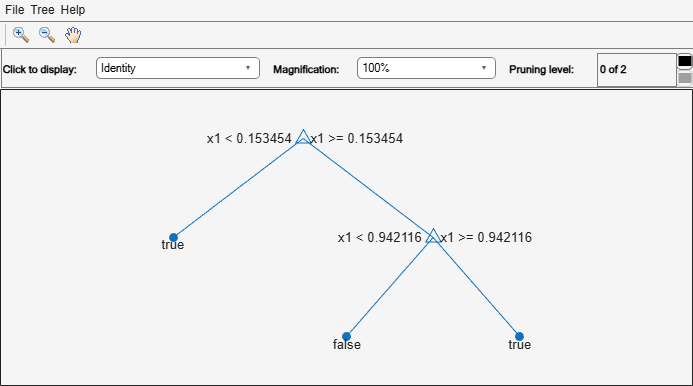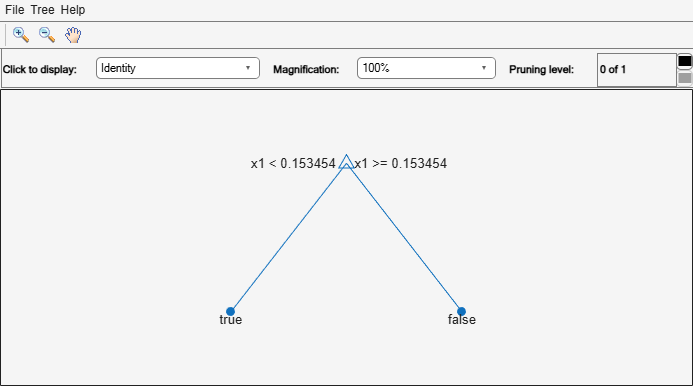# resubPredict

Predict resubstitution labels of classification tree

## Syntax

``label = resubPredict(tree)``
``[label,posterior] = resubPredict(tree)``
``[label,posterior,node] = resubPredict(tree)``
``[label,posterior,node,cnum] = resubPredict(tree)``
``[label,___] = resubPredict(tree,Name,Value)``

## Description

example

````label = resubPredict(tree)` returns the labels `tree` predicts for the data `tree.X`. `label` is the predictions of `tree` on the data that `fitctree` used to create `tree`.```

example

````[label,posterior] = resubPredict(tree)` returns the posterior class probabilities for the predictions.```
````[label,posterior,node] = resubPredict(tree)` returns the node numbers of `tree` for the resubstituted data.```
````[label,posterior,node,cnum] = resubPredict(tree)` returns the predicted class numbers for the predictions.```

example

````[label,___] = resubPredict(tree,Name,Value)` returns resubstitution predictions with additional options specified by one or more `Name,Value` pair arguments.```

## Examples

collapse all

Find the total number of misclassifications of the Fisher iris data for a classification tree.

```load fisheriris tree = fitctree(meas,species); Ypredict = resubPredict(tree); % The predictions Ysame = strcmp(Ypredict,species); % True when == sum(~Ysame) % How many are different?```
```ans = 3 ```

Load Fisher's iris data set. Partition the data into training (50%)

`load fisheriris`

Grow a classification tree using the all petal measurements.

```Mdl = fitctree(meas(:,3:4),species); n = size(meas,1); % Sample size K = numel(Mdl.ClassNames); % Number of classes```

View the classification tree.

`view(Mdl,'Mode','graph');`The classification tree has four pruning levels. Level 0 is the full, unpruned tree (as displayed). Level 4 is just the root node (i.e., no splits).

Estimate the posterior probabilities for each class using the subtrees pruned to levels 1 and 3.

`[~,Posterior] = resubPredict(Mdl,'SubTrees',[1 3]);`

`Posterior` is an `n`-by- `K`-by- 2 array of posterior probabilities. Rows of `Posterior` correspond to observations, columns correspond to the classes with order `Mdl.ClassNames`, and pages correspond to pruning level.

Display the class posterior probabilities for iris 125 using each subtree.

`Posterior(125,:,:)`
```ans = ans(:,:,1) = 0 0.0217 0.9783 ans(:,:,2) = 0 0.5000 0.5000 ```

The decision stump (page 2 of `Posterior`) has trouble predicting whether iris 125 is versicolor or virginica.

Classify a predictor `X` as true when `X < 0.15` or `X > 0.95`, and as false otherwise.

Generate 100 uniformly distributed random numbers between 0 and 1, and classify them using a tree model.

```rng("default") % For reproducibility X = rand(100,1); Y = (abs(X - 0.55) > 0.4); tree = fitctree(X,Y); view(tree,"Mode","graph")```Prune the tree.

```tree1 = prune(tree,"Level",1); view(tree1,"Mode","graph")```The pruned tree correctly classifies observations that are less than 0.15 as `true`. It also correctly classifies observations from 0.15 to 0.95 as `false`. However, it incorrectly classifies observations that are greater than 0.95 as `false`. Therefore, the score for observations that are greater than 0.15 should be about 0.05/0.85=0.06 for `true`, and about 0.8/0.85=0.94 for `false`.

Compute the prediction scores (posterior probabilities) for the first 10 rows of `X`.

```[~,score] = resubPredict(tree1); [score(1:10,:) X(1:10)]```
```ans = 10×3 0.9059 0.0941 0.8147 0.9059 0.0941 0.9058 0 1.0000 0.1270 0.9059 0.0941 0.9134 0.9059 0.0941 0.6324 0 1.0000 0.0975 0.9059 0.0941 0.2785 0.9059 0.0941 0.5469 0.9059 0.0941 0.9575 0.9059 0.0941 0.9649 ```

Indeed, every value of `X` (the right-most column) that is less than 0.15 has associated scores (the left and center columns) of 0 and 1, while the other values of `X` have associated scores of approximately 0.91 and 0.09. The difference (score of 0.09 instead of the expected 0.06) is due to a statistical fluctuation: there are 8 observations in `X` in the range (0.95,1) instead of the expected 5 observations.

`sum(X > 0.95)`
```ans = 8 ```

## Input Arguments

collapse all

Classification tree, specified as a `ClassificationTree` object. Use the `fitctree` function to create a classification tree object.

### Name-Value Arguments

Specify optional pairs of arguments as `Name1=Value1,...,NameN=ValueN`, where `Name` is the argument name and `Value` is the corresponding value. Name-value arguments must appear after other arguments, but the order of the pairs does not matter.

Before R2021a, use commas to separate each name and value, and enclose `Name` in quotes.

Example: ```[~,posterior] = resubPredict(tree,'SubTrees',[1 3]);```

Pruning level, specified as a vector of nonnegative integers in ascending order or `"all"`.

If you specify a vector, then all elements must be at least `0` and at most `max(tree.PruneList)`. `0` indicates the full, unpruned tree and `max(tree.PruneList)` indicates the completely pruned tree (i.e., just the root node).

If you specify `"all"`, then `resubPredict` operates on all subtrees (in other words, the entire pruning sequence). This specification is equivalent to using `0:max(tree.PruneList)`.

`resubPredict` prunes `tree` to each level indicated in `Subtrees`, and then estimates the corresponding output arguments. The size of `Subtrees` determines the size of some output arguments.

To invoke `Subtrees`, the properties `PruneList` and `PruneAlpha` of `tree` must be nonempty. In other words, grow `tree` by setting `Prune="on"`, or by pruning `tree` using `prune`.

Example: `Subtrees="all"`

Data Types: `single` | `double` | `char` | `string`

## Output Arguments

collapse all

Response `tree` predicts for the training data, returned as a vector or matrix. `label` is the same data type as the training response data `tree.Y`.

If the `Subtrees` name-value pair argument contains `m`>`1` entries, then `label` is returned as a matrix with `m` columns, each of which represents the predictions of the corresponding subtree. Otherwise, `label` is returned as a vector.

Posterior probabilities for classes `tree` predicts, returned as a matrix or array.

If the `Subtrees` name-value argument is a scalar or is missing, `posterior` is an `n`-by-`k` matrix, where `n` is the number of rows in the training data `tree.X`, and `k` is the number of classes.

If `Subtrees` contains `m`>`1` entries, `posterior` is an `n`-by-`k`-by-`m` array, where the matrix for each `m` gives posterior probabilities for the corresponding subtree.

Node numbers of `tree` where each data row resolves, returned as a vector or matrix.

If the `Subtrees` name-value argument is a scalar or is missing, `node` is a numeric column vector with `n` rows, the same number of rows as `tree.X`.

If `Subtrees` contains `m`>`1` entries, `node` is a `n`-by-`m` matrix. Each column represents the node predictions of the corresponding subtree.

Class numbers that `tree` predicts for resubstituted data, returned as a vector or matrix.

If the `Subtrees` name-value argument is a scalar or is missing, `cnum` is a numeric column vector with `n` rows, the same number of rows as `tree.X`.

If `Subtrees` contains `m`>`1` entries, `cnum` is a `n`-by-`m` matrix. Each column represents the class predictions of the corresponding subtree.

collapse all

### Posterior Probability

The posterior probability of the classification at a node is the number of training sequences that lead to that node with this classification, divided by the number of training sequences that lead to that node.

For an example, see Posterior Probability Definition for Classification Tree.

## Version History

Introduced in R2011a